# Vector variable in TMC classification

Dear experts,
I have a vector branch in my root file and I parse it to the TMVA classification (1). The code run fine and the vector variable looks fine (2). If this makes sense, I wonder how can I parse this “vector variable” to the reader? using (3) does not work with the “vector” type.
Regards

(1)

(2)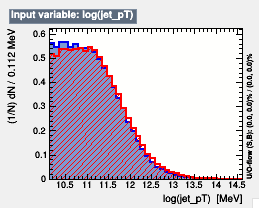(3)
vector<float> r_jet_pT;

Dear experts,
any ideas?
Regards

Hi,

In (1) TMVA will consider only the first entry of the vector. (The variable is added as a float).

This means you have to make the reader understand the same thing by

``````TMVA::Reader *reader = new TMVA::Reader( “!Color:!Silent” );
Float_t r_jet_pT;
``````

Cheers,
Kim

Dear Kim,

• I run the same code with the vector of pt (the leading pt is the 1st value in the vector) and the leading pt variable. I checked that the vector always correspond to the leading pt, but TMVA give me different output, here are the log file:
1. vector: http://calpas.web.cern.ch/calpas/log_vec

you can see that the ROC is 0.727 for the vector and 0.719 for the leading pt variable. Depending on how I configure the DNN these ROC are significantly different.

Here are the response and input variable:
3) vector: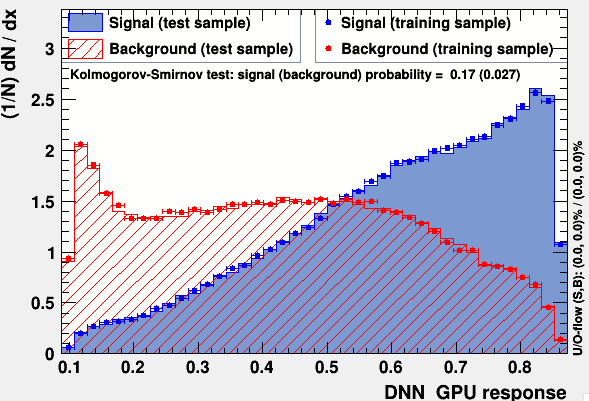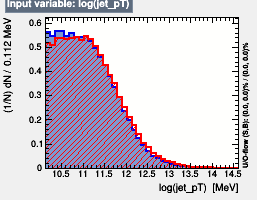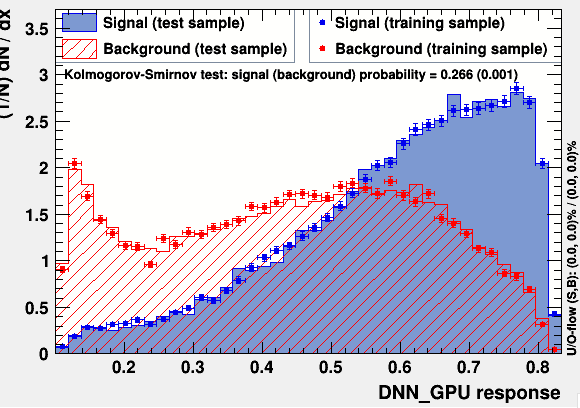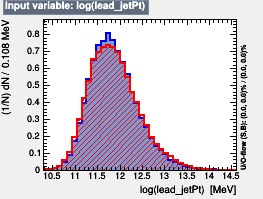Do you see where is coming these differences?

Regards

Hi,

TMVA will include concatenate all entries in an array variable, keeping all other non-array variables constant. Having two variables, one scalar (`x`) and one vector (`y`) with a length of two would create the following TMVA events

``````(x_0, y_{0, 0})
(x_0, y_{0, 1})
(x_1, y_{1, 0})
(x_1, y_{1, 1})
``````

where the first index indicates sample id and the second indexes into the vector.

This means you probably will want to add the variable as

``````dataloader->AddVariable( “vec_jet_pT_0 := vec_jet_pT” “MeV”, ‘F’ );
``````

or similar. This would be equivalent (according to you description) to

``````dataloader->AddVariable( “vec_jet_pT_0 := lead_jet_pT” “MeV”, ‘F’ );
``````

One can of course then add the other entries as

Cheers,
Kim

Dear Kialbert,
does it make sense if I just use:

I mean what the code does if I use that? It seems to work.

Regards

Hi,

1. If your variable `vector_pT` can be seen as a single variable then this makes sense.

2. If you want your classifier to be able to make decisions based on `vector_pT` and `vector_pT` separately, then you’d have to do something different.

So, when you plot `vector_pT`, if you always flatten the 2-d vector into a 1-D one I’d say 1) is fine. Otherwise be carefulCheers,
Kim

Dear Kialbert,
my problem is that I do not know what the TMVA code does, I wonder if an expert know what the code does if I use: dataloader->AddVariable( “vector_pT”, “vector_pT”, “MeV”, ‘F’ );
I mean I’m just running the tools. When I used “vector_pT” I see much better separation than if I use " vector_pT", so I wonder what can explain this difference? does it use all the values in the vector, or only one of them…?
Regards

Hi,

This is what I’m trying, and unfortunately failing, to explain. Let me try with a different approach.`lead_jet_pT` is a property of an event while `vec_jet_pT` contains data for individual jets. TMVA tries to be helpful when you add a vector variable and assumes that you want to do classification on the individual jets (as opposed to the whole physics event). So it creates new `TMVA::Event`'s for each entry in the vector; A vector with length 5 would, instead of 1 `TMVA::Event`, generate 5 `TMVA::Event`'s.

The `TMVA::Reader` is not directly aware of this behaviour, so you would have to replicate it manually to have consistent results between training/testing (`TMVA::Factory`) and application (`TMVA::Reader`).

Something along the lines of the code snippet below would be necessary.

``````int x = 0;
float vec_pT = 0.;

tree = GetTree();
// Note we are not using `AddVariable("vec_pT")` here.

for (ievent = 0; i < tree->GetEntries(); ++ievent) {
// This will get the correct value of `x`
tree->GetEntry(ievent);
for (ijet = 0; i < numJets; ++ijet) {
// This would get, in turn, each of the jet pt's and feed them into tmva
// Warning: there are probably better ways of doing this, and I'm not even sure
// it works as is written here, but I hope you understand the idea.
vec_pT = *(static_cast<float *>(tree->FindLeaf("vec_pT")->GetValuePointer()) + ijet);
std::cout << "My prediction is: " << prediction << " for event " << ievent*numJets + ijet << std::endl;
}
}
``````

I hope it’s a bit more clear now.

Cheers,
Kim

Dear Kim,

in TMVAClassification.C decided to used (1). To parse that to the reader, I did (2), but I got the error message (3). Do you see what is wrong? You can see the entire code here (4).
Regards

(1)

(2)

float r_jet_pT_0;

itree->SetBranchStatus(“jet_pT”, 1);
vector *jet_pT;

for (Long64_t ievt=0; ievtGetEntries(); ievt++) {
r_jet_pT_0 = jet_pT;
}

(3)

Dear experts,
any idea?
Regards

In your code the variable `jet_pT` is declared as a pointer to vector of float `vector<float> *jet_pT;`. Changing your assignment from `r_jet_pT_0 = jet_pT;` to `r_jet_pT_0 = (*jet_pT);` should do the trick.

(That is instead of setting `r_jet_pT_0` to the first vector of floats in `jet_pT`, set it to the first element of the pointed-to vector.)

Cheers,
Kim